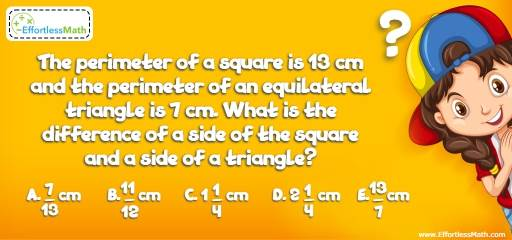# Geometry Puzzle – Challenge 73

Time for another great math challenge for those who enjoy solving mathematics and critical thinking challenges.## Challenge:

The perimeter of a square is 13 cm and the perimeter of an equilateral triangle is 7 cm. What is the difference of a side of the square and a side of a triangle?

A- $$\frac{7}{13}$$ cm

B- $$\frac{11}{12}$$ cm

C- $$1\frac{1}{4}$$ cm

D- $$2\frac{1}{4}$$ cm

E- $$\frac{13}{7}$$ cm

### The Absolute Best Book to challenge your Smart Student!

The perimeter of a square is 13cm. Therefore, one side of the square is $$\frac{13}{4}$$ cm.
The perimeter of the equilateral triangle is 7cm. Therefore, one side of the triangle is $$\frac{7}{3}$$ cm.
The difference of a side of the square and a side of a triangle is:
$$\frac{13}{4} – \frac{7}{3} = \frac{39-28}{12} = \frac{11}{12}$$ cm

### What people say about "Geometry Puzzle – Challenge 73 - Effortless Math: We Help Students Learn to LOVE Mathematics"?

No one replied yet.

X
30% OFF

Limited time only!

Save Over 30%

SAVE $5 It was$16.99 now it is \$11.99